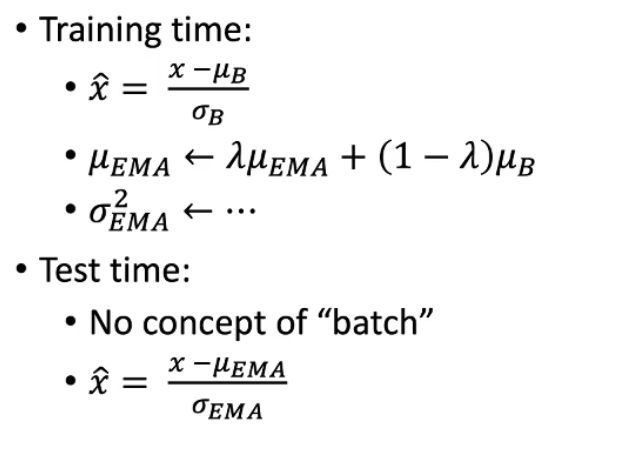o
osc_g8254g7s

# <center>Devils in BatchNorm</center>

Batch Norm的训练和测试具有不一致性，测试时vanilla BN方法是更新一个exponential moving average，也就是图中的$u_{EMA}$• 为什么可以训练和测试不一致？ DropOut和Data Augmentation也是这样——可以理解为训练是在测试的基础上加噪声，测试是训练的平均。

• BN什么时候会失败？ 当$\mu_{EMA}$，$\sigma_{EMA}$不接近$\mu_{B}$和$\sigma_{B}$时
1. 当EMA计算不合理
2. 当$\mu_{B}$，$\sigma_{B}$不稳定时 - 不能很好地近似 a）数据不稳定 b）不稳定的模型
• EMA计算不合理的情况 $$\mu_{E M A} \leftarrow \lambda \mu_{E M A}+(1-\lambda) \mu_{B}, \sigma_{E M A}^{2} \leftarrow \cdots$$
1. $\lambda$过小，EMA
2. $\lambda$过大，需要很多次迭代
3. 不稳定的模型或最后N次迭代中不稳定的数据

• EMA不合理之处
1. 总是有偏置的
2. 数据的分布总是在变化
3. 并不是真的平均
• 解决方案：Precise BatchNorm 最早来源于ResNet 实现： · Cheap Precise BN:继续使用EMA但是使用大的$\lambda$，把模型固定，forward很多（比如1000次）迭代 · 先算前一层的PreciseBN，用这个再算下一层PreciseBN

## BN在训练/微调上的坑

### Normalization batch size

1. Norm batch size不一定等于SGD batch size，受显卡显存的限制
2. 一个batch中，均值和方差是有噪声的——上面提到训练的均值和方差可看作在测试的基础上加噪声，若一个batch中有一个异常sample就带来噪声
• 如何增大Normalization batch size？
1. Sync BatchNorm/Cross-GPU BN 其实现是采用all-reduce $2 \times C$ elements。 overhead也很小。在各个框架上都有实现。
2. Virtual BatchNorm 使用很多只为了前向的图片，不会显著增加显存，但是会增加时间。 唯一好处是可控，适用于reasearch和analysis。
• 如何减小Normalization batch size？ Ghost BN 其实现是在一个batch中分离

• NBS特别小时的解决方案 Batch Renormalization。 训练: $\hat{x}=\frac{x-\mu_{B}}{\sigma_{B}} \times$ stop gradient $(r)+$ stop gradient $(d)$ 测试: $\hat{x}=\frac{x-\mu_{E M A}}{\sigma_{E M A}}$ $r, d$ pushes $\mu_{B}, \sigma_{B}$ similar to $\mu_{E M A}, \sigma_{E M A}$ Reduce noise $&$ inconsistency Need to tune the limit on $r, d$

## BN在数据分布的分布

1. 多域学习
2. 对抗训练
3. fine-tuning 一些解决的tricks：
4. 训练时——为各个domain做Seperate BN
5. 训练/微调时——Frozen BN（Sync BN没出现前使用，一般不全部用于train from scratch，用于fine-tune或是train时模型的末端）

#### GAN中遇到的real/fake分布

1. decoder(real_batch,training=True)
2. decoder(fake_batch,training=True,update_ema=False)# don't update EMA或decoder(fake_batch,training=False)# use EMA during training

#### batch本来的设计就来源于相关源

1. two-stage目标检测器中batch本身就有来自同一张图片的patch组成 -> 解决：Group Norm
2. 视频理解

## BN在实现上的坑

PyTorch中momentum的0.1是别人的0.9，而且及其需要注意track_running_stats的使用； TensorFlow中EMA的更新不是在层计算的同时发生，新手容易忘记更新EMA更新的操作加入到训练中，解决方法是使用tensorpack.models.BatchNorm；

## TensorFlow实现BN

def batch_norm(x,beta,gamma,phase_train,scope='bn',decay=0.9,eps=1e-5):
with tf.variable_scope(scope):
# beta = tf.get_variable(name='beta', shape=[n_out], initializer=tf.constant_initializer(0.0), trainable=True)
# gamma = tf.get_variable(name='gamma', shape=[n_out],
#                         initializer=tf.random_normal_initializer(1.0, stddev), trainable=True)
batch_mean,batch_var = tf.nn.moments(x,[0,1,2],name='moments')
ema = tf.train.ExponentialMovingAverage(decay=decay)

def mean_var_with_update():
ema_apply_op = ema.apply([batch_mean,batch_var])
with tf.control_dependencies([ema_apply_op]):
return tf.identity(batch_mean),tf.identity(batch_var)
# identity之后会把Variable转换为Tensor并入图中，
# 否则由于Variable是独立于Session的，不会被图控制control_dependencies限制

mean,var = tf.cond(phase_train,
mean_var_with_update,
lambda: (ema.average(batch_mean),ema.average(batch_var)))
normed = tf.nn.batch_normalization(x, mean, var, beta, gamma, eps)
return normed


# 总结

1. 使用哪个$\mu, \sigma ?$ $\mu_{B}$, $\sigma_{B}$ ; $\mu_{E M A}$, $\sigma_{E M A}$ ; Batch ReNorm

2. 如何计算$\mu_{B}$, $\sigma_{B}$: Per-GPU BN,Sync BN,Ghost BN,Virtual BN

3. 是否更新$\mu_{E M A}$, $\sigma_{E M A}$With $\mu_{B}$, $\sigma_{B}$: YES,NO,Separate BN

4. 测试/微调时用什么: EMA,Precise BN,Adaptive BN,Frozen BN

o

### osc_g8254g7s#### 暂无文章

40分钟前
9
0
Python的__init__和self是做什么的？ - What __init__ and self do on Python?

javail

15
0
OSChina 周五乱弹 —— 你大妈还是你大妈

Osc乱弹歌单（2020）请戳（这里） 【今日歌曲】 @watergood：是时候分享一波我的这张纯音乐歌单了，过去的五年多时间里，我陆陆续续地把听到的好听的纯音乐添加了进去，目前一共65首，相信总...

25
0

13
0
ftp-ftps-sftp的关系

Ftp FTP 是File Transfer Protocol（文件传输协议）的英文简称，而中文简称为“文传协议”。用于Internet上的控制文件的双向传输。同时，它也是一个应用程序（Application）。基于不同的操作...

12
0